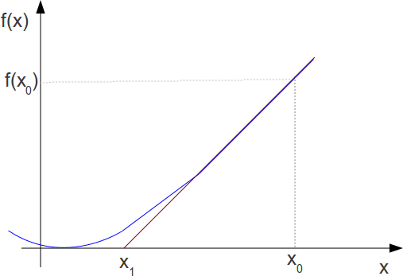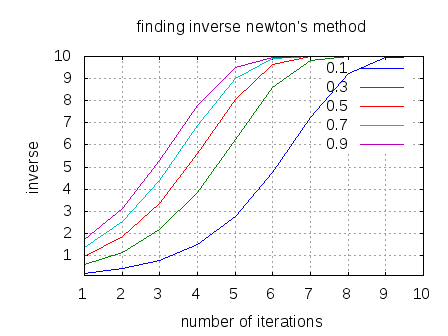(1 votes, average: 5.00 out of 5)Loading ...

# Newton’s method to find square root, inverse

by on December 25, 2011

Some of us would have used Newton’s method (also known as Newton-Raphson method) in some form or other. The method has quite a bit of history,  starting with the Babylonian way of finding the square root and later over centuries reaching the present recursive way of finding the solution. In this post, we will describe Newton’s method and apply it to find the square root and the inverse of a number.

## Geometrical interpretationWe know that the derivative of a function at  is the slope of the tangent (red line) at  i.e.,

.

Rearranging, the intercept of the tangent at x-axis is,

.

From the figure above, can see that the tangent (red line) intercepts the x-axis at  which is closer to  the  where compared to . Keep on doing this operation recursively, and it converges to the zero of the function OR in another words the root of the function.

In general for iteration, the equation is :

.

## Finding square root

Let us, for example try to use this method for finding the square root of D=100. The function to zero out in the Newton’s method frame work  is,

, where .

The first derivative is

.

The recursive equation is,

.

Matlab code snippet

clear ; close all;
D = 100; % number to find the square root
x = 1; % initial value
for ii = 1:10
fx = x.^2 - D;
f1x = 2*x;
x = x - fx/f1x;
x_v(ii) = x;
end
x_v' =
50.5000000000000
26.2400990099010
15.0255301199868
10.8404346730269
10.0325785109606
10.0000528956427
10.0000000001399
10.0000000000000
10.0000000000000
10.0000000000000

We can see that the it converges within around 8 iterations. Further, playing around with the initial value,

a) if we start with initial value of x = -1, then we will converge to -10.

b) if we start with initial value of x = 0, then we will not converge

and so on…

## Finding inverse (division)

Newton’s method can be used to find the inverse of a variable D. One way to write the function to zero out is, but we soon realize that this does not work as we need know in the first place.

Alternatively the function to zero out can be written as,

.

The first derivative is,

.

The equation in the recursive form is,

.

Matlab code snippet

clear ; close all;
D = .1; % number to find the square root
x = [.1:.2:1]; % initial value
for ii = 1:10
fx = (1./x) - D;
f1x = -1./x.^2;
x = x - fx./f1x;
x_v(:,ii) = x;
end
plot(x_v');
legend('0.1', '0.3', '0.5', '0.7', '0.9');
grid on; xlabel('number of iterations'); ylabel('inverse');
title('finding inverse newton''s method');

The following plot shows the convergence of inverse computation to the right value for different values of for this example matlab code snippet.Figure : convergence of inverse computation

## Finding the minima of a function

To find the minima of a function, we to find where the derivative of the function becomes zero i.e.  .

Using Newton’s method, the recursive equation becomes :

.

## Thoughts

We have  briefly gone through the Newton’s method and its applications to find the roots of a function, inverse, minima etc. However, there are quite a few aspects which we did not go over, like :

a) Impact of the initial value on the convergence of the function

b) Rate of the convergence

c) Error bounds of the converged result

d) Conditions where the convergence does not happen

and so on…

Hoping to discuss those in another post…## References

Wiki on Newton’s method

D id you like this article? Make sure that you do not miss a new article by subscribing to RSS feed OR subscribing to e-mail newsletter. Note: Subscribing via e-mail entitles you to download the free e-Book on BER of BPSK/QPSK/16QAM/16PSK in AWGN.ali November 7, 2012 at 3:12 pm